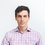# Partition functions, physics, and DNA search engines

To summarize our work, we've found an object $f(z)$, which we can define for any probability distribution $p(m)$, that serves as a wellspring for all quantities of interest associated with the distribution. It makes calculations more transparent, eases more advanced manipulations, and frees up mental computing power for more interesting tasks.

Our object $f(z)$, defined as $\sum\limits_m p(m)z^m$, works as it does because we exploit the derivative properties of $z^m$ as a tool. However, because we chose $z^m$, our derivatives are a little ugly.

For instance, to find the mean, we take a single derivative, $f'(z)$, but for the mean squared value we add two derivatives together, $f''(1) + f'(1)$. As we try to find higher moments, the dance gets less and less appetizing.

This situation arises because each time we take a derivative of $z^m$, we bring down a new function of $m$: $m, m-1,\ldots, 1$ rather than $m,m,\ldots$. Things would be nicer if this could be arranged.

An alternative choice that gets the job done is $e^{zm}$, so that $f(z) = \sum\limits_m e^{zm}p(m)$.

In our voting model, we'd instead have $f(z) = \left(p_Ae^{zm} + p_B\right)^N$, and find the simpler relations

$\langle \hat{A} \rangle = \frac{\partial}{\partial z} f(z) \bigg|_{z=0}$

and

$\langle \hat{A}^2 \rangle = \frac{\partial^2}{\partial z^2} f(z) \bigg|_{z=0}$

The object that results from this choice is called the moment generating function and it is a powerful tool. It is so powerful in fact that a foundational domain of theoretical physics, statistical mechanics, has been constructed around its use.

In a system that is close to thermodynamic equilibrium, the likelihood of the system occupying a particular state $s$, of energy $E_s$ is proportional to the factor $\displaystyle e^{-E_s/k_BT} = e^{-E_s/\beta}$.

The engine of statistical mechanics is a kind of moment generating function, a sum over the likelihood of all energetic states, $E_s$, known as the partition function, $Z$. It encapsulates all the statistical properties of a physical system and allows us to obtain them through imaginative manipulations.

Concretely:

$Z = \sum\limits_s e^{-E_s/k_BT}$

The probability of occupying the state is given by the relative likelihood, normalized by the partition function (the sum of all likelihoods):

$p(E_S) = \frac{e^{-E_s/k_BT}}{Z}$

What this translates to is that, all else being equal (temperature, volume, pressure, etc.), a system tends to occupy states of lower energy.

Though slightly more intimidating than before, we find that useful quantities such as the average energy can be found through derivatives of $Z$

\begin{aligned} \langle E \rangle &= \frac{1}{Z}\sum\limits_s e^{-E_s\beta}E_s \\ &= \frac{1}{Z} \sum\limits_s \frac{\partial}{\partial \beta}e^{-E_s\beta} \\ &= \frac{1}{Z} \frac{\partial}{\partial \beta} \sum\limits_s e^{-E_s\beta} \\ &= \frac{1}{Z} \frac{\partial}{\partial \beta} Z \\ &= \frac{\partial}{\partial \beta} \log Z \end{aligned}

where $\beta = \left(k_BT\right)^{-1}$

Physics and politics should never be equated. We can, nevertheless, obtain our higher moments ($\langle E^2 \rangle - \langle E \rangle ^2$) through derivatives similar ($\frac{\partial^2}{\partial \beta^2} \log Z$) to what we used in the voting model.

The success of the partition function in physics has catapulted it to use in areas as far flung as neuroscience, computation biology, and the study of natural language.

Consider a particular DNA molecule (top strand in diagrams below) diffusing in liquid with millions of other DNA molecules. It is more likely to be found in a state of maximum pair bondingthan in a state with multiple mismatchesThe reason for this is that each stable bond the DNA molecule makes with its partner molecule (A:T, or G:C) lowers the energy of interaction, contributing a factor $e^{-E_s\beta}$.

On the other hand, each mismatch (A:G, C:T, etc.) produces a high energy bulge, contributing a factor $e^{-E_b\beta}$.

The bulges are expensive ($e^{-E_s\beta} \gg e^{-E_b\beta}$ ) to maintain in the face of lower energy alternatives and, so, DNA molecules are most likely to be found with their most stable partners. The probability distribution of the various binding events can be captured through a suitably constructed partition function describing the energetics.

Hopefully, you can now see the divine beauty of the generating function approach.

Let's wrap things up, using the partition function to fold a simple protein.Note by Josh Silverman
7 years, 3 months ago

This discussion board is a place to discuss our Daily Challenges and the math and science related to those challenges. Explanations are more than just a solution — they should explain the steps and thinking strategies that you used to obtain the solution. Comments should further the discussion of math and science.

When posting on Brilliant:

• Use the emojis to react to an explanation, whether you're congratulating a job well done , or just really confused .
• Ask specific questions about the challenge or the steps in somebody's explanation. Well-posed questions can add a lot to the discussion, but posting "I don't understand!" doesn't help anyone.
• Try to contribute something new to the discussion, whether it is an extension, generalization or other idea related to the challenge.

MarkdownAppears as
*italics* or _italics_ italics
**bold** or __bold__ bold
- bulleted- list
• bulleted
• list
1. numbered2. list
1. numbered
2. list
Note: you must add a full line of space before and after lists for them to show up correctly
paragraph 1paragraph 2

paragraph 1

paragraph 2

[example link](https://brilliant.org)example link
> This is a quote
This is a quote
    # I indented these lines
# 4 spaces, and now they show
# up as a code block.

print "hello world"
# I indented these lines
# 4 spaces, and now they show
# up as a code block.

print "hello world"
MathAppears as
Remember to wrap math in $$ ... $$ or $ ... $ to ensure proper formatting.
2 \times 3 $2 \times 3$
2^{34} $2^{34}$
a_{i-1} $a_{i-1}$
\frac{2}{3} $\frac{2}{3}$
\sqrt{2} $\sqrt{2}$
\sum_{i=1}^3 $\sum_{i=1}^3$
\sin \theta $\sin \theta$
\boxed{123} $\boxed{123}$

Sort by:

Dude, nice. I feel like not enough people have recognized this amazing note.

- 7 years, 3 months ago

Maybe we did, didn't we?

- 6 years, 7 months ago

wwwwwwwwoooooooowwwwwwwww! great work sir !

- 4 years, 4 months ago